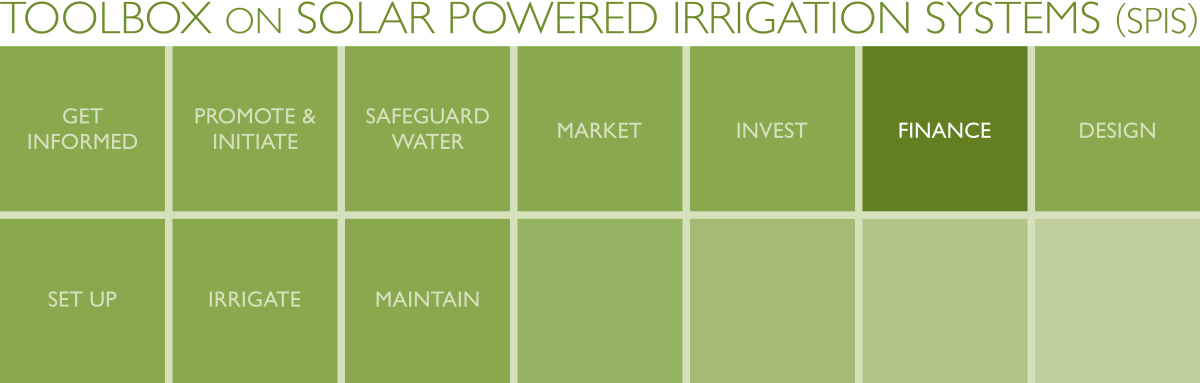# SPIS Toolbox - Finance - Annex## Annex - Collection of Formulae (Finances)

AVERAGE CASH FLOW*

 *Definition: The “cash flow” is the incoming and outgoing cash of a business. Expenses (costs) are considered as negative cash flows and revenues as positive ones.

Formula: (Revenue-R –Operating Expenses-C) = Cf. = Cash flow

PAYBACK PERIOD* (PP)

 *Definition: The payback period is the length of time required to recover the cost of an investment.

Formula: I/(R-C) = PP = Payback Period

I=Initial investment (CAPEX)

C=Average annual operating expenses (OPEX), excluding depreciation

R=Average annual revenue

(R-C) = Cf. = Cash flow

NET PRESENT VALUE* (NPV)

 *Definition: The “Net Present Value” or NPV determines the present worth of an investment by discounting the cash inflows and cash outflows generated by this investment over its life span. For the determination of the NPV you need to define the expected life span of the investment as well as a discount factor, which might be near to the interest rate on deposits. You could also use the NPV for comparison of alternative investment options.

Formula

 r= Discount factor S= Salvage ValueI= Initial investment costt= years counting from base yearn= lifetime of project (panels)
 INTERNAL RATE OF RETURN* (IRR)
 *Definition: The “Internal Rate of Return” or IRR gives the discount rate over the lifespan of a capital investment; i.e. the profit rate generated by a certain investment (amount) over its lifespan. By calculating IRR of a project you can answer the question whether the money is well spent or if less risky investment alternatives might be more profitable in the long run, e.g. putting the money on a bank account to get interest on it.

Formula